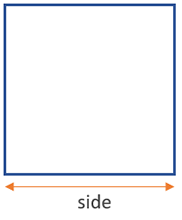# Area of a Square Calculator

Use this calculator to easily calculate the area of a square given the length of its side in any metric: mm, cm, meters, km, inches, feet, yards, miles...

### Calculation results

9 cm2 Square area
Share calculator:

Embed this tool:
get code

## Area of a square formula

The formula for the area of a square is side2, as seen in the figure below:The solution to the equation is straightforward multiplication and this is the formula used in our area of a square online calculator. The result will be in whatever metric you did the measurement in, but squared: square mm, square cm, square dm, square meters or square inches, square feet, square yards, square miles etc.

## How to calculate the area of a square?

The area of a square is one of the easiest to calculate in that it requires just one measurement of the square to be known - its side. You then multiply it by itself to get to the area, so the formula used in this area of a square calculator is just as simple.

The simplicity of the square is why it is usually one of the first shapes that geometry students become familiar with. In real life measurements, like in construction, engineering, landscaping, etc. we rarely deal with square areas and surfaces - they are more often rectangular in shape. Still it is a good teaching device as it contains the rules for solving many other shapes.

This is because a square can be interpreted as a special case of a rhombus (equal sides and opposite equal angles), a kite (two pairs of adjacent equal angles), a trapezoid (one pair of opposite sides are parallel), a parallelogram (all opposite sides are parallel), and, of course, a rectangle, in which the opposite sides are equal in length and all of its angles are 90°. Therefore, a square combines the properties of all of these shapes: diagonals bisect at 90°, diagonals bisect the square angles, diagonals are equal, the sides are equal, opposite sides are equal, all angles are equal (90°). Learning to calculate the area of a square is thus a precursor for learning how to calculate the areas of more advanced shapes.

## Example: find the area of a square

The only measurement needed to find the area of a square figure is its side. Since all sides are equal it does not matter which side is measured. Then simply multiply the measurement by itself to get the area.

For example, if the side of a square pool is 10 yards, then the pool area is 10 x 10 = 100 square yards. If one side of a square room is 12 feet, then its area is 12 x 12 = 144 square feet.

#### Cite this calculator & page

If you'd like to cite this online calculator resource and information as provided on the page, you can use the following citation:
Georgiev G.Z., "Area of a Square Calculator", [online] Available at: https://www.gigacalculator.com/calculators/area-of-square-calculator.php URL [Accessed Date: 27 Mar, 2023].